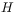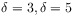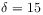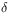• Abscence of hyperscaling violations for phase transitions with positive specific heat exponent
Sie sind hier: ICP » R. Hilfer » Publikationen

# Abscence of hyperscaling violations for phase transitions with positive specific heat exponent

###### Abstract.

Finite size scaling theory and hyperscaling are analyzed in the ensemble limit which differs from the finite size scaling limit. Different scaling limits are discussed. Hyperscaling relations are related to the identification of thermodynamics as the infinite volume limit of statistical mechanics. This identification combined with finite ensemble scaling leads to the conclusion that hyperscaling relations cannot be violated for phase transitions with strictly positive specific heat exponent. The ensemble limit allows to derive analytical expressions for the universal part of the finite size scaling functions at the critical point. The analytical expressions are given in terms of general-functions, scaling dimensions and a new universal shape parameter. The universal shape parameter is found to characterize the type of boundary conditions, symmetry and other universal influences on critical behaviour. The critical finite size scaling functions for the order parameter distribution are evaluated numerically for the casesandwhereis the equation of state exponent. Using a tentative assignment of periodic boundary conditions to the universal shape parameter yields good agreement between the analytical prediction and Monte-Carlo simulations for the twodimensional Ising model. Analytical expressions for critical amplitude ratios are derived in terms of critical exponents and the universal shape parameters. The paper offers an explanation for the numerical discrepancies and the pathological behaviour of the renormalized coupling constant in mean field theory. Low order moment ratios of difference variables are proposed and calculated which are independent of boundary conditions, and allow to extract estimates for a critical exponent.

###### Key words and phrases:
PACS: 05.20.-y 05.70.-a 05.70.Fh 05.70.Jk